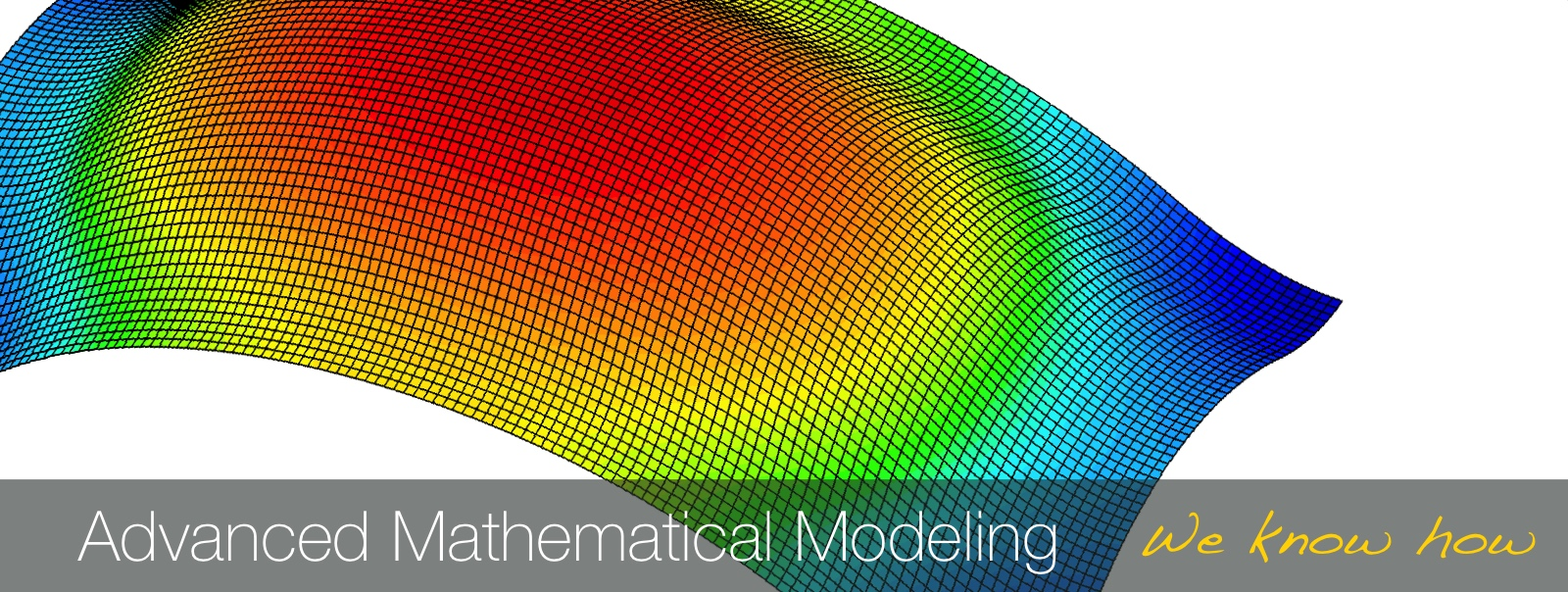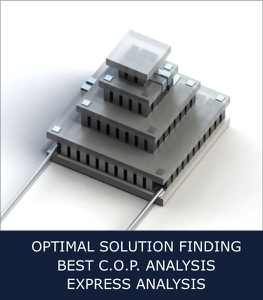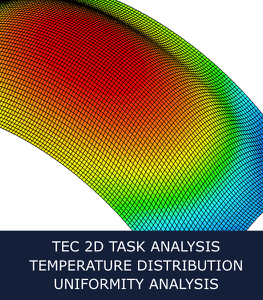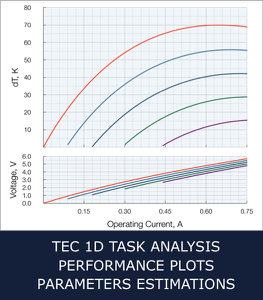Precise mathematical estimations and analysis are the key to success in optimal solution developments. It it especially important in a field of thermoelectricity. The mathematical model to be as close to real application conditions as possible. TEC Microsystems GmbH has a professional team, specializing in accurate mathematical analysis and precise estimations. With more than 15 years of experience we take into account every single aspect and provide the most accurate and precise estimations for optimal thermoelectric solution. The developed mathematical core model is ultimately precise and quick. It has been polishing over the years and now allows to provide ultra-fast search for the most optimal thermoelectric cooling solution from millions of available types. Our laboratory experiments with real TE module performance check proof the deviation between estimations and real values with now more than 5%.

Advanced Mathematical modeling and quick search for optimal solution- In depth analysis of optimal thermoelectric solutions for various application conditions

- Scenario-based comparison of cooling and heating regime performance of Peltier-modules

- Optimal operations points for specified Peltier-modules under external conditions

- Dependency charts of all relevant variables for specified application conditions (e.g.  [∆T, I, U, Q]max, C.O.P., passive heat loads, maximal Rth, etc.)